# Difference between revisions of "Tutte polynomial"

Letbe a matroid with rank functionon the ground set. The Tutte polynomial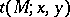ofis defined by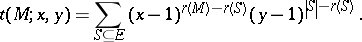One also writes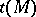forwhen no confusion can arise about the values ofand. There are several equivalent reformulations of; the most useful such expression is recursive, using the matroid operations of deletion and contraction:

T0) If, then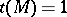;

T1) Ifis an isthmus, then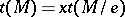;

T2) Ifis a loop, then;

T3) Ifis neither an isthmus nor a loop, then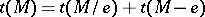.

Some standard evaluations of the Tutte polynomial are:

a)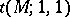is the number of bases of;

b)is the number of independent subsets of;

c)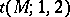is the number of spanning subsets of;

d)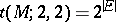.

When a matroidis constructed from other matroids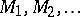, it is frequently possible to computefrom the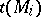. The most fundamental structural result of this kind concerns the direct sum of matroids: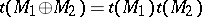. Another fundamental structural property of the Tutte polynomial is its transparent relationship with matroid duality: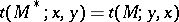. Many related results can be found in [a3].

The Tutte polynomial has many significant connections with invariants in graph theory. Of central importance is the relationship betweenand the chromatic polynomial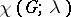of a graph(cf. also Graph colouring). Ifis a graph having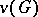vertices and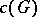connected components, then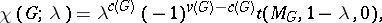where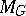is the cycle matroid of(cf. also Matroid). Thus, whenis planar (cf. Graph, planar),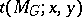simultaneously carries information concerning proper colourings ofalong with proper colourings of its dual graph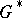. This is the reason W.T. Tutte referred to it as a dichromatic polynomial in his foundational work in this area [a14]. The polynomial was generalized from graphs to matroids by H.H. Crapo [a5] and T.H. Brylawski [a2].

Other invariants which can be obtained from the Tutte polynomial include the beta invariant, the Möbius function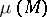, and the characteristic polynomial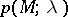of a matroid. See [a16] for more information about these invariants.

## Applications

The Tutte polynomial has applications in various areas, some of which are given below. For an extensive introduction, see [a4].

Acyclic orientations: Let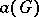denote the number of acyclic orientations of a graph. Then R. Stanley [a13] proved that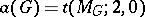. A variety of related evaluations appear in [a9].

The critical problem of Crapo and G.-C. Rota [a7]: Letbe a rank-matroid which is represented overby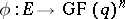and letbe a positive integer. Then the number of-tuples of linear functionals onwhich distinguishequals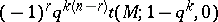. See [a12] for extensions of the critical problem.

Coding theory: Letbe a linear code overwith code-weight polynomial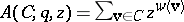, where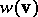is the weight of the code word(see also Coding and decoding). Then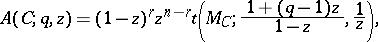where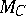is the matroid associated with the code. Many standard results in coding theory have interpretations using the Tutte polynomial [a4].

Hyperplane arrangements: The number of regions in a central arrangement of hyperplanes is given by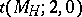, where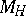is the matroid associated with the arrangement. Many generalizations of this result appear in [a15].

Combinatorial topology: The independent subsets of a matroid form a shellable complex complex. If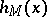is the shelling polynomial associated with this complex, then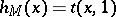and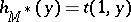. This result is analogous to the dichromatic interpretation for planar graphs. See [a1] for more information on how the Tutte polynomial is associated to simplicial complexes.

Statistical mechanics, network reliability and knot theory: Supposeis a probabilistic matroid, i.e., each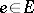has an independent probability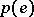of successful operation. The formula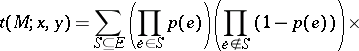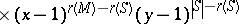then produces a probabilistic Tutte polynomial. In this more general situation,is the reliability of, i.e., the probability that a randomly chosen subset spans. Related Tutte polynomials have applications in statistical mechanics and network reliability [a5] and knot theory [a11].

Greedoids: Whenis a greedoid (cf. also Greedy algorithm), the expansion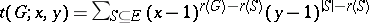remains valid. The recursion of T3) takes a slightly different form, however: Ifis feasible, then. There are many combinatorial structures which admit a greedoid rank function in a natural way, but do not possess a meaningful matroid structure. For example, if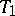andare rooted trees (cf. also Tree), then computing the Tutte polynomial in this way givesif and only ifandare isomorphic as rooted trees [a8].

In general, it is-hard (cf.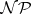) to compute the Tutte polynomial of a graph or a matroid [a10]. Certain evaluations are computable in polynomial-time for certain classes of matroids, however. For example, the number of spanning trees of a graph can be calculated in polynomial-time by the matrix-tree theorem; since this number equals, this evaluation is tractable.

How to Cite This Entry:
Tutte polynomial. Encyclopedia of Mathematics. URL: http://encyclopediaofmath.org/index.php?title=Tutte_polynomial&oldid=38555
This article was adapted from an original article by G. Gordon (originator), which appeared in Encyclopedia of Mathematics - ISBN 1402006098. See original article